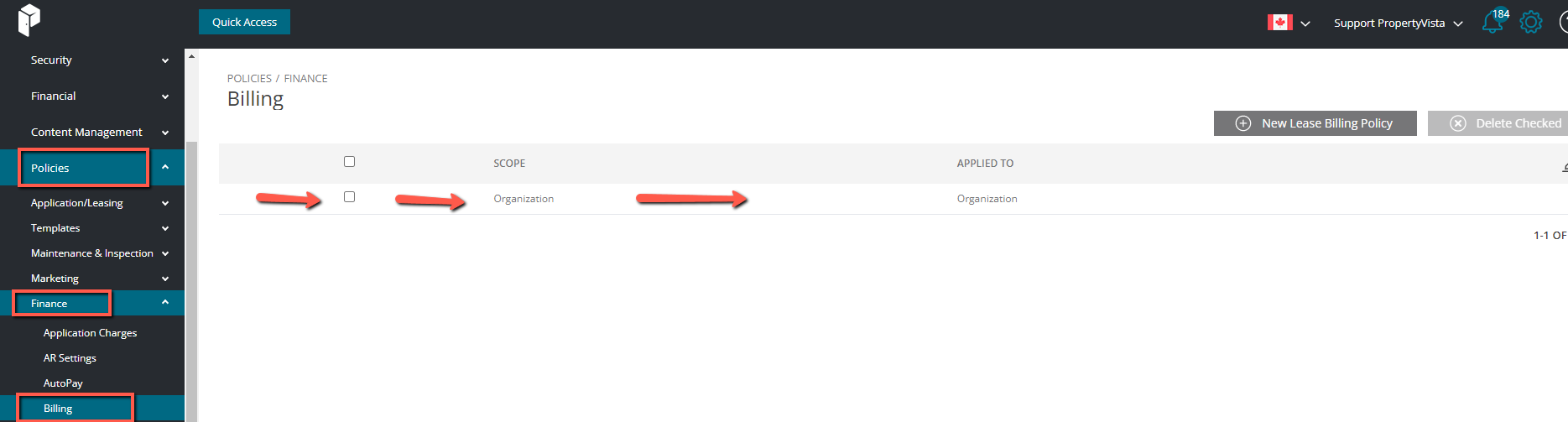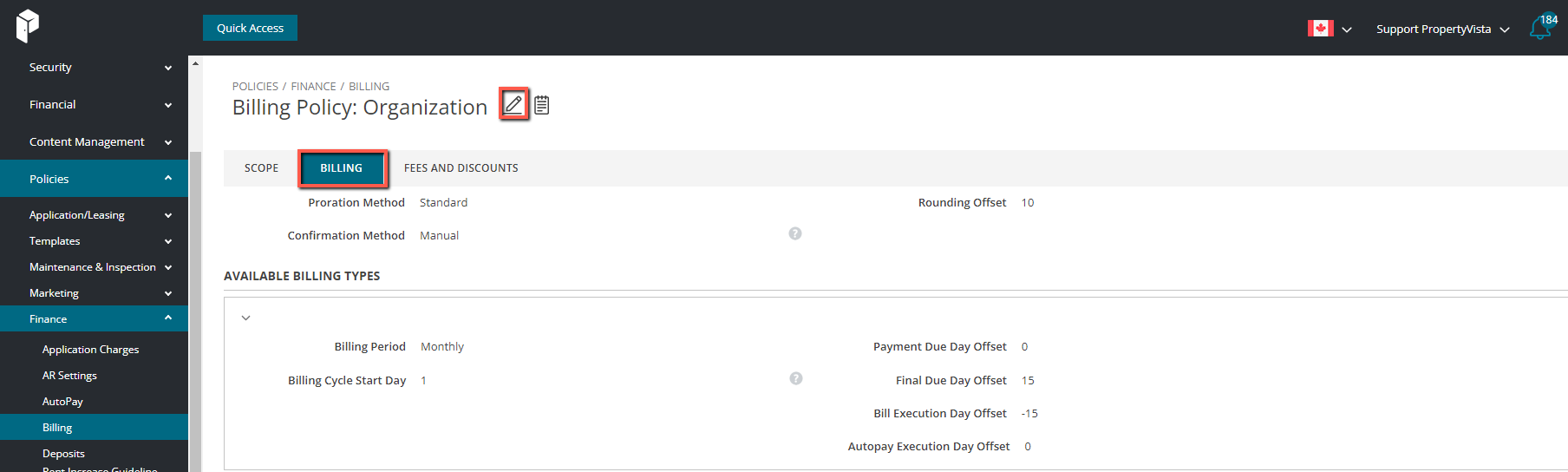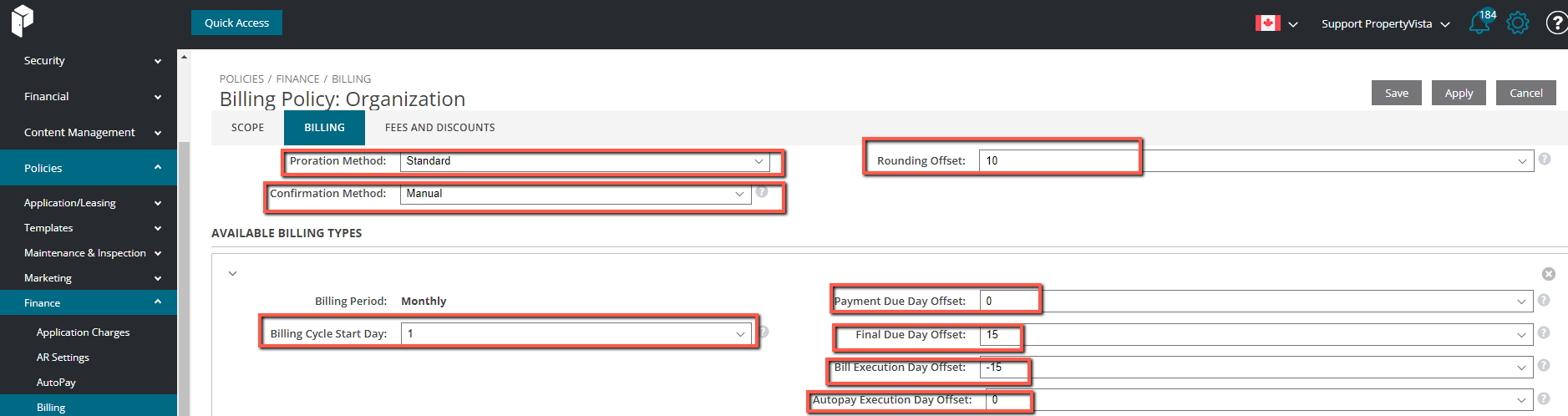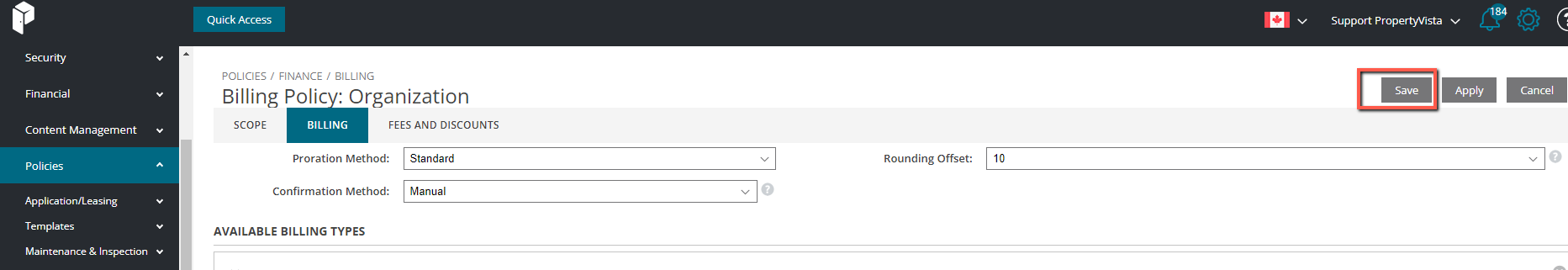# Set-up Prorate Policy Rules

The Billing Policy allows you to set prorate rules for your leasing.

Step 1: Navigate to Administration Policies Finance Billing → select a policy ( In this case, Organization) → Billing (beside Scope).Step 2: Select the Billing tabSelect the pencil to editKindly refer below to information relating to each category.Category Dropdown Option(s) Details Proration Method None No prorate (the tenant will be charged the full month of rent for the prorated month) E.g if the move-in date is August 15th, the tenant is charged the total monthly amount for August Actual Prorate is calculated based on monthly rent price, actual days in the prorated month that the tenant will be living there, and the actual number of days in the prorated month.   Calculation: (monthly rent / # of days in the month) x # of actual days occupying the unit = prorated rent amount Standard Prorate is calculated based on the monthly rent price, 30 days in the prorated month and the actual number of days of the lease in the prorated month.   Calculation: (monthly rent / 30 days) x # of days occupying the unit = prorated rent amount 30-Day Month Prorate is calculated based on the monthly lease price, 30 days in the prorate month, and the number of days of the lease in the prorated month between the start date and the 30th of the month. Calculation: (monthly rent / 30 days) x # of days of the lease in the prorated month between the start date and 30th of the month = prorated rent amount Note: For 31 day month, if the start date is 30th or 31st, the number of days is 1. For 31 day month, if the start date is 29th, the number of days is 2. For 28 day month, if the start date is the 25th, the number of days is 4. E.g. Count of Days (Aug 8 - Aug 31) = 23 days Annual (Standard, 365 Days) Prorate is calculated based on annualized lease price, the actual number of days in the prorated month, and 365 days in a year Calculation: (total rent for the year / 365 days in a year) x # of days occupying the unit = prorated rent amount (Actual, Leap Year Check) Prorate is calculated based on annualized lease price, the actual number of days in the prorated month, and 365 days in a year (OR 366 days where it is a leap year) Non-Leap Year Calculation: (total rent for the year / 365 days in a year) x # of days occupying the unit = prorated rent amount   Leap Year Calculation: If it is a leap year, the calculation will be (total rent for the year / 366 days in a year) x # of days occupying the unit = prorated rent amount Choose the rounding offset values (the rounding offset defines the lease term end date) Number (1-31) Day of rounding offset from the start date of the billing cycle. Example: If "10" is Selected applications created with a move-in date before the 10th would start their term in the given month, while applications after the 10th would be rounded up to the following month to start their term Number 0 Rounding to the last day of the previous billing cycle (or previous end of the month, if Billing Cycle Start Day is set to 1) Not Set No rounding (future functionality) Billing Cycle Start Day Click + Add under the Available Billing Types. The Billing Cycle Start Day can be any day of the month. For example, if set to 1, it is equal to the 1st of the month. It can also be set as the same as Lease Start Day Payment Due Offset 0-15 Choose "0" if the payment is due the same day as the Billing Cycle. If rent is due after you can select the offset amount here Bill Execution Day Offset -15 to -1 How many days before the next billing period should the system create the Bill/Invoice for the Tenants Autopay Execution Day Offset -15 to 15 When to run the AutoPayments relative to the lease cycle

Step 3: Press Save